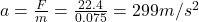## A dart gun consists of a horizontal spring with k = 52 Newtons/m that is compressed 43 the gun is cocked. What is the initial accelera

Question

A dart gun consists of a horizontal spring with k = 52 Newtons/m that is compressed 43
the gun is cocked. What is the initial acceleration of a 75-g dart when the gun is fired?

in progress 0
3 months 2021-08-12T23:48:17+00:00 1 Answers 1 views 0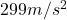Explanation:

When a spring is compressed, the force exerted by the spring is given by: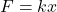where

k is the spring constant

x is the compression of the spring

In this problem we have:

k = 52 N/m is the spring constant

x = 43 cm = 0.43 m is the compression

Therefore, the force exerted by the spring on the dart is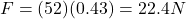Now we can apply Newton’ second law of motion to calculate the acceleration of the dart: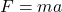where

F = 22.4 N is the force exerted on the dart by the spring

m = 75 g = 0.075 kg is the mass of the dart

a is its acceleration

Solving for a,